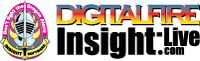•The secret to cool bodies and glazes is a lot of testing.
•The secret to know what to test is material and chemistry knowledge.
•The secret to learning from testing is documentation.
•The place to test, do the chemistry and document is an account at https://insight-live.com
•The place to get the knowledge is https://digitalfire.com

Sign-up at https://insight-live.com today.

# FM Fireclay

 Oxide Analysis Formula CaO 0.16% 0.013 MgO 0.69% 0.080 K2O 1.88% 0.093 Na2O 0.04% 0.003 P2O5 0.06% 0.002 TiO2 1.32% 0.077 Al2O3 21.96% 1.000 SiO2 64.63% 4.995 Fe2O3 1.29% 0.037 LOI 7.97 Oxide Weight 427.46 Formula Weight 464.48

## XML for Import into INSIGHT

<?xml version="1.0" encoding="UTF-8"?> <material name="FM Fireclay" descrip="" searchkey="" loi="7.97" casnumber="70694-09-6"> <oxides> <oxide symbol="CaO" name="Calcium Oxide, Calcia" status="" percent="0.160" tolerance=""/> <oxide symbol="MgO" name="Magnesium Oxide, Magnesia" status="" percent="0.690" tolerance=""/> <oxide symbol="K2O" name="Potassium Oxide" status="" percent="1.880" tolerance=""/> <oxide symbol="Na2O" name="Sodium Oxide, Soda" status="" percent="0.040" tolerance=""/> <oxide symbol="P2O5" name="Phosphorus Pentoxide" status="" percent="0.060" tolerance=""/> <oxide symbol="TiO2" name="Titanium Dioxide, Titania" status="" percent="1.320" tolerance=""/> <oxide symbol="Al2O3" name="Aluminum Oxide, Alumina" status="U" percent="21.960" tolerance=""/> <oxide symbol="SiO2" name="Silicon Dioxide, Silica" status="" percent="64.630" tolerance=""/> <oxide symbol="Fe2O3" name="Iron Oxide, Ferric Oxide" status="" percent="1.290" tolerance=""/> </oxides> <volatiles> <volatile symbol="LOI" name="Loss on Ignition" percent="7.970" tolerance=""/> </volatiles> </material>